# Over Lesson 4 3 Over Lesson 4 3

• Slides: 22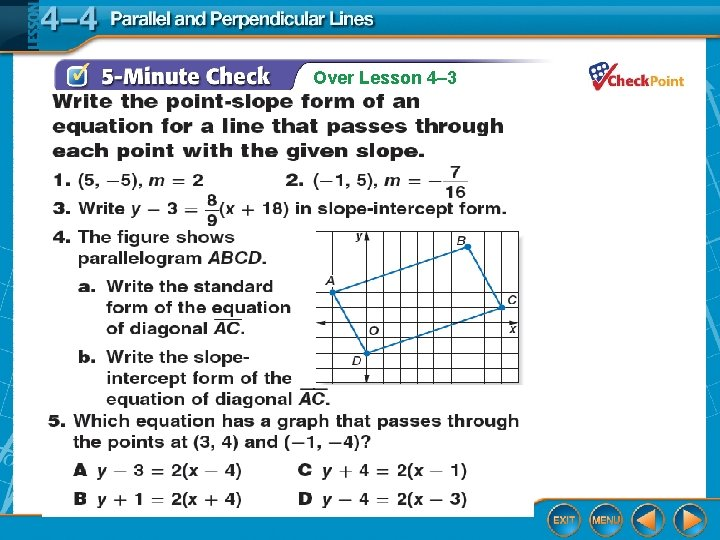Over Lesson 4– 3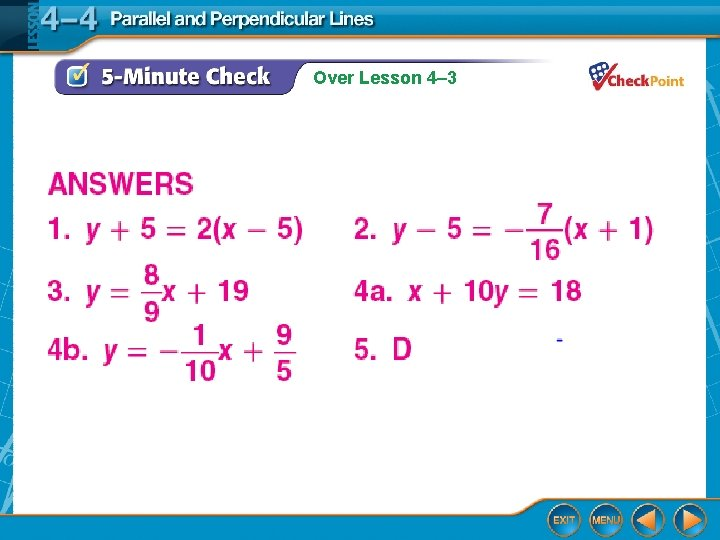Over Lesson 4– 3Parallel and Perpendicular Lines Lesson 4 -4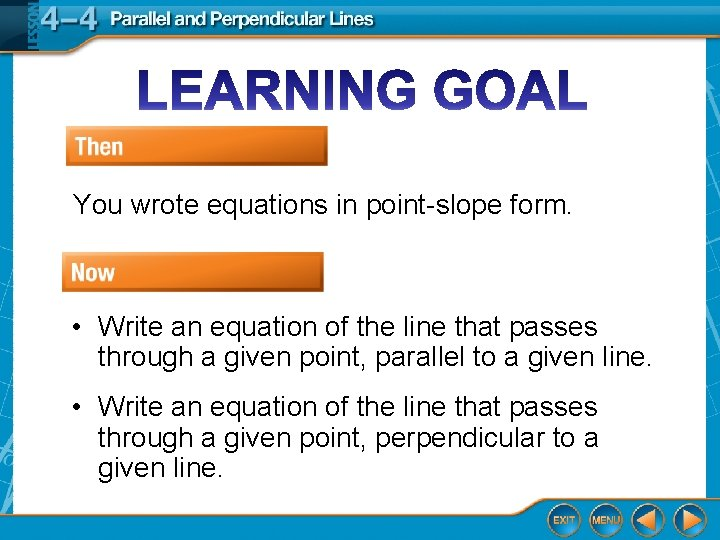You wrote equations in point-slope form. • Write an equation of the line that passes through a given point, parallel to a given line. • Write an equation of the line that passes through a given point, perpendicular to a given line.Slopes of perpendicular lines are opposite, inverses of each other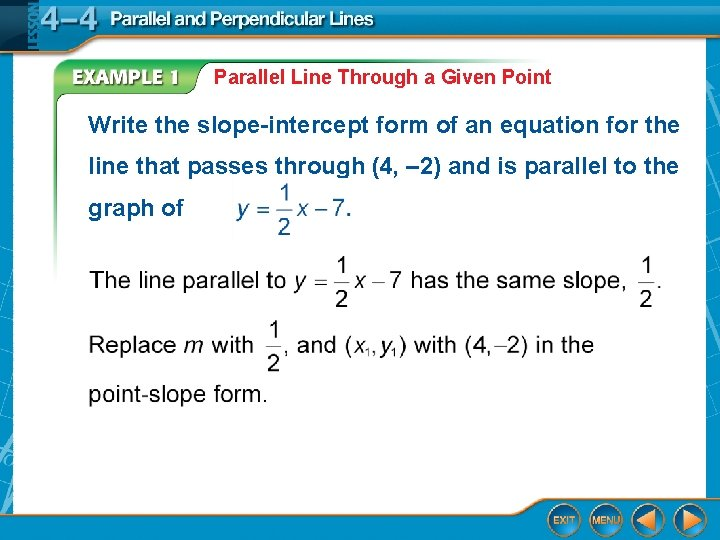Parallel Line Through a Given Point Write the slope-intercept form of an equation for the line that passes through (4, – 2) and is parallel to the graph of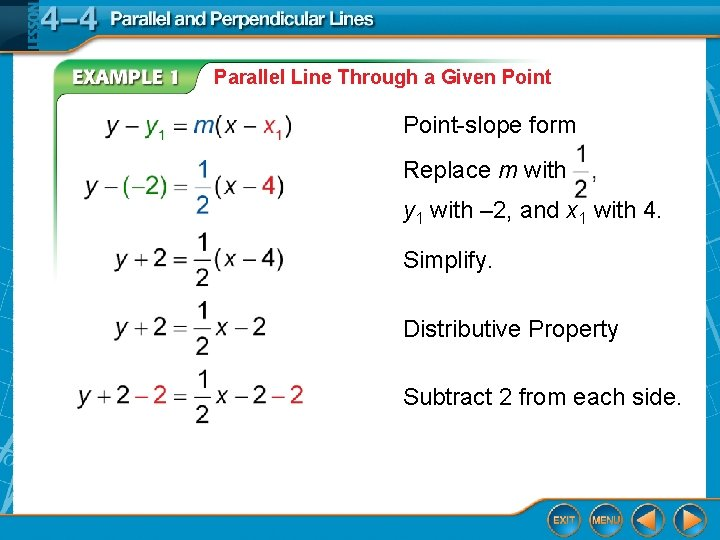Parallel Line Through a Given Point-slope form Replace m with y 1 with – 2, and x 1 with 4. Simplify. Distributive Property Subtract 2 from each side.Parallel Line Through a Given Point Write the equation in slope-intercept form. Answer: The equation is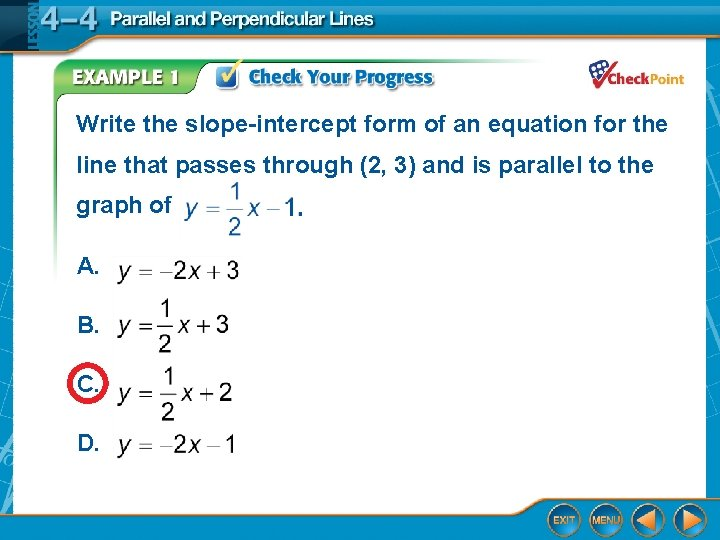Write the slope-intercept form of an equation for the line that passes through (2, 3) and is parallel to the graph of A. B. C. D.Slopes of Perpendicular Lines A. GEOMETRY The height of a trapezoid is the length of a segment that is perpendicular to both bases. In trapezoid ARTP, RT and AP are bases. Can EZ be used to measure the height of the trapezoid? Explain.Slopes of Perpendicular Lines Find the slope of each segment. Answer: The slope of RT and AP is 1 and the slope of EZ is – 7. Since 1(– 7) ≠ – 1, EZ is not perpendicular to RT and AP. So, it cannot be used to measure the height of ARTP.Slopes of Perpendicular Lines B. GEOMETRY The height of a trapezoid is the length of a segment that is perpendicular to both bases. In trapezoid ARTP, RT and AP are bases. Are the bases parallel? Answer: Yes, both RT and AP have a slope of 1.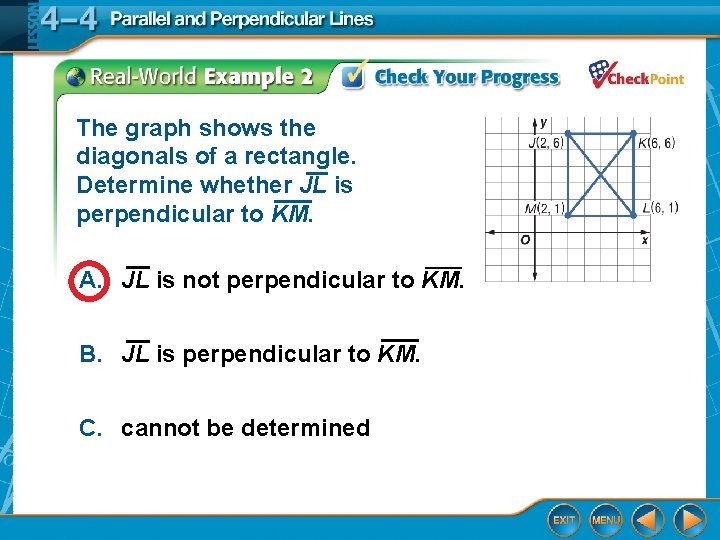The graph shows the diagonals of a rectangle. Determine whether JL is perpendicular to KM. A. JL is not perpendicular to KM. B. JL is perpendicular to KM. C. cannot be determinedParallel or Perpendicular Lines Determine whether the graphs of 3 x + y = 12, and 2 x – 6 y = – 5 are parallel or perpendicular. Explain. Graph each line on a coordinate plane.Parallel or Perpendicular Lines Answer: From the graph, you can see that is parallel to 2 x – 6 y = – 5. They are parallel because they have equal slopes. 3 x + y = 12 is perpendicular to them both because the product of their slopes, and – 3, is – 1.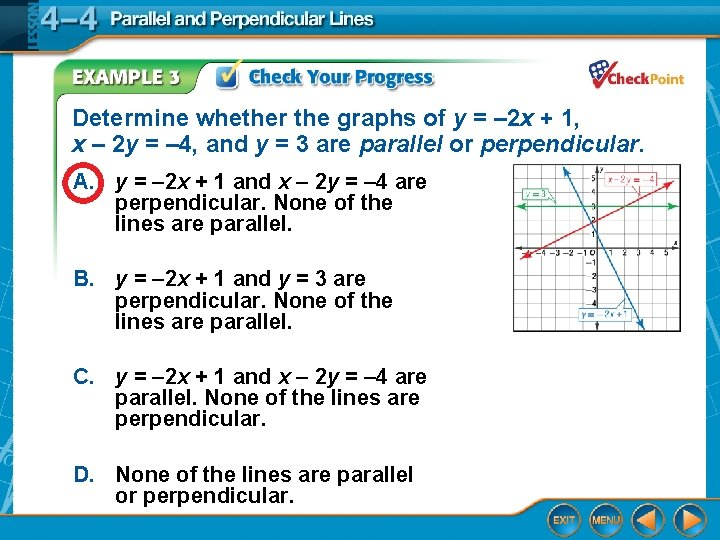Determine whether the graphs of y = – 2 x + 1, x – 2 y = – 4, and y = 3 are parallel or perpendicular. A. y = – 2 x + 1 and x – 2 y = – 4 are perpendicular. None of the lines are parallel. B. y = – 2 x + 1 and y = 3 are perpendicular. None of the lines are parallel. C. y = – 2 x + 1 and x – 2 y = – 4 are parallel. None of the lines are perpendicular. D. None of the lines are parallel or perpendicular.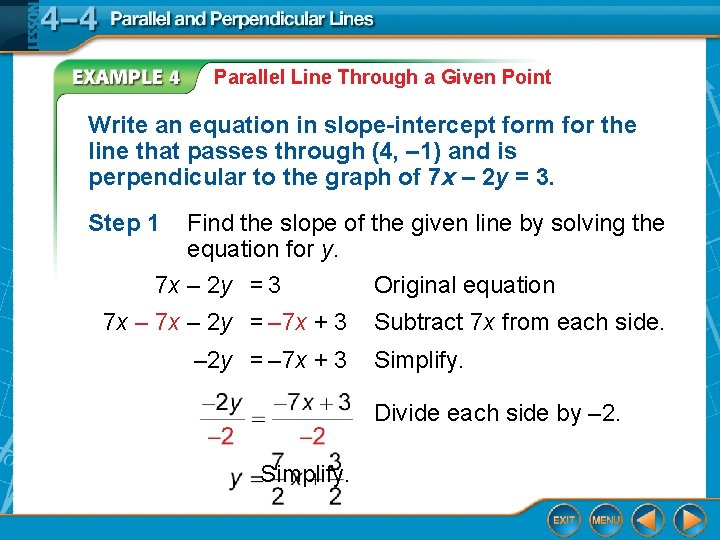Parallel Line Through a Given Point Write an equation in slope-intercept form for the line that passes through (4, – 1) and is perpendicular to the graph of 7 x – 2 y = 3. Step 1 Find the slope of the given line by solving the equation for y. 7 x – 2 y = 3 Original equation 7 x – 2 y = – 7 x + 3 Subtract 7 x from each side. Simplify. Divide each side by – 2. Simplify.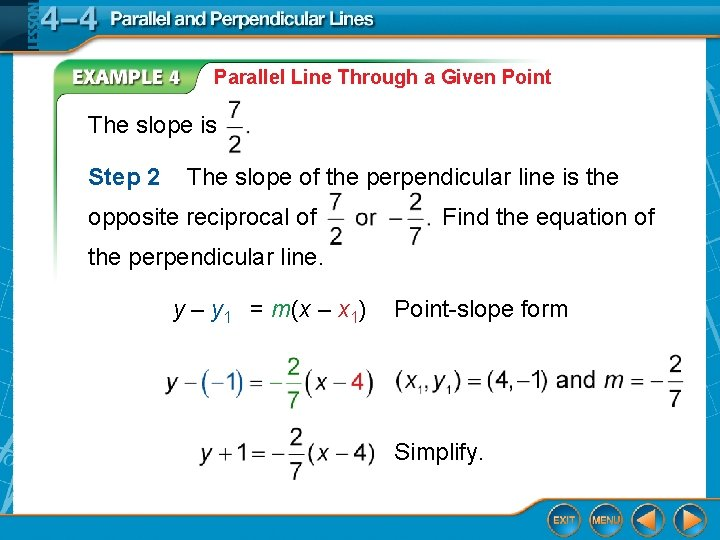Parallel Line Through a Given Point The slope is Step 2 The slope of the perpendicular line is the opposite reciprocal of Find the equation of the perpendicular line. y – y 1 = m(x – x 1) Point-slope form Simplify.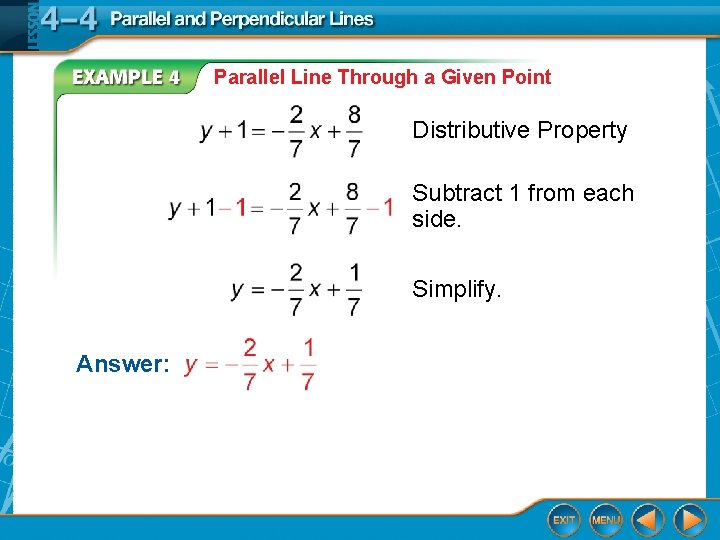Parallel Line Through a Given Point Distributive Property Subtract 1 from each side. Simplify. Answer: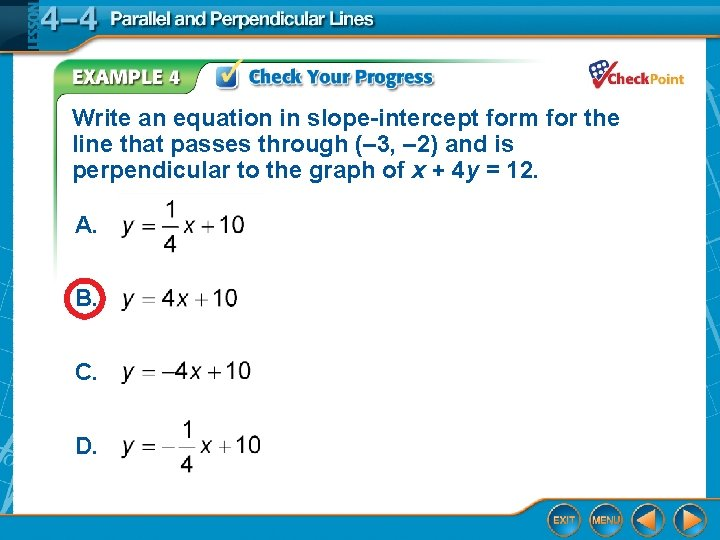Write an equation in slope-intercept form for the line that passes through (– 3, – 2) and is perpendicular to the graph of x + 4 y = 12. A. B. C. D.Homework Page 243 #11 -47 odd